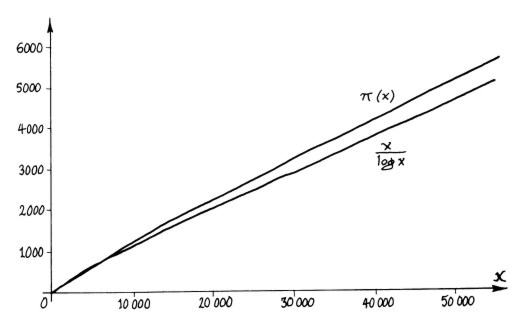In 1896, de la Valee Poussin and Hadamard simultaneously proved what had been suspected for several decades, and what is now known as the prime number theorem:In words, the (discontinuous) prime counting function π(x) is asymptotic to the (smooth) logarithmic function x/log x. This means that the ratio of π(x) to x/log x can be made arbitrarily close to 1 by considering large enough x. Hence x/log x provides an approximation of the number of primes less than or equal to x, and if we take sufficiently large x this approximation can be made as accurate as we would like (proportionally speaking – very simply, as close to 100% accuracy as is desired). The original proofs of the prime number theorem suggested other, better approximations. In the above graph we see that x/log x, despite being asymptotic to π(x), is far from being the smooth function which π(x) suggests when we zoom out – there is plenty of room for improving the approximation. These improvements turn out to be greatly revealing. << previous      beginning      next >>